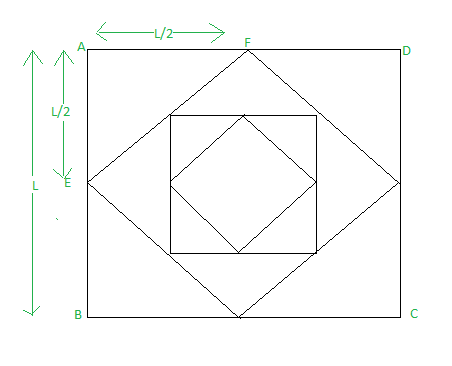Related Articles
Area of squares formed by joining mid points repeatedly
• Difficulty Level : Medium
• Last Updated : 01 Oct, 2020

Given a square having a side of length L. Another square form inside the first square by joining midpoint of the side of the first square. Now 3rd square is form inside 2nd one by joining midpoints of the side of the 2nd square and so on.
You have 10 squares inside each other. and you are given side length of largest square.you have to find the area of these 10 squares.

Examples:

Input : L = 5, n = 10
Output :49.9512

Input : L = 2, n = 2
Output :7.99219



This article can be understand using diagram that depicts the whole problem.From the diagram-
Area of outermost square will be given by L^2.
Area of 2nd square-As length of 2nd square can be calculate using Pythagoras theorem., side for this square will be \\Area of 3rd square-
Similarly area of third square can be calculated, we getArea of 4th square-
Similarly area of forth square can be calculated, we getHence this form Geometric progression, having first term as L^2 and common ratio = 1/2.

Now we have to find sum of first n terms of this GP.

## C++

 // C++ program to find Area of squares// formed by joining mid points repeatedly#include #define ll long long intusing namespace std;int main(){    double L = 2, n = 10;     double firstTerm = L * L;    double ratio = 1 / 2.0;     // apply GP sum formula    double sum = firstTerm * (pow(ratio, 10) - 1) / (ratio - 1);     cout << sum << endl;     return 0;}

## Java

 // Java program to find Area of squares// formed by joining mid points repeatedlyimport java.util.*;import java.lang.*;import java.io.*;import java.lang.Math;/* Name of the class has to be "Main" only if the class is public. */class GFG{    public static void main (String[] args) throws java.lang.Exception    {         double L = 2, n = 10;       double firstTerm = L * L;    double ratio = 1 / 2.0;       // apply GP sum formula    double sum = firstTerm * (Math.pow(ratio, 10) - 1) / (ratio - 1);       System.out.println(sum) ;         }}//  This code is contributed by Shrikant13

## Python 3

 # Python3 program to find Area of squares# formed by joining mid points repeatedly if __name__=='__main__':    L = 2    n = 10     firstTerm = L * L    ratio = 1 / 2 # apply GP sum formula    sum = firstTerm * ((ratio**10) - 1) / (ratio - 1)     print(sum) # This code is contributed by# Sanjit_Prasad

## C#

 // C# program to find area of squares// formed by joining mid points repeatedlyusing System; // Name of the class has to be// "Main" only if the class is public.class GFG{     public static void Main(string[] args){    double L = 2;    //double n = 10;         double firstTerm = L * L;    double ratio = 1 / 2.0;         // Apply GP sum formula    double sum = firstTerm *                 (Math.Pow(ratio, 10) - 1) /                          (ratio - 1);         Console.Write(Math.Round(sum, 5));}} // This code is contributed by rutvik_56
Output:
7.99219



Time Complexity: O(1)

Attention reader! Don’t stop learning now. Get hold of all the important DSA concepts with the DSA Self Paced Course at a student-friendly price and become industry ready.

My Personal Notes arrow_drop_up